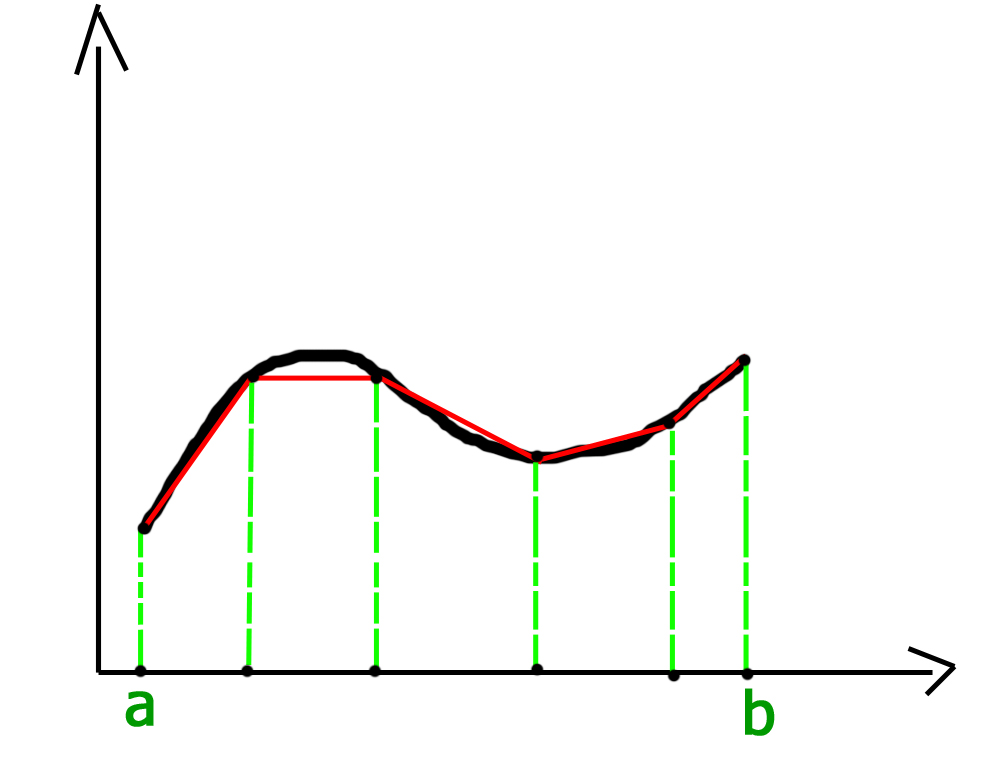# Trapezoidal Rule for Approximate Value of Definite Integral

In the field of numerical analysis, Trapezoidal rule is used to find the approximation of a definite integral. The basic idea in Trapezoidal rule is to assume the region under the graph of the given function to be a trapezoid and calculate its area.
It follows that:For more accurate results the domain of the graph is divided into n segments of equal size as shown below:Grid spacing or segment size h = (b-a) / n.
Therefore, approximate value of the integral can be given by:## C++

 // C++ program to implement Trapezoidal rule  #include     // A sample function whose definite integral's  // approximate value is computed using Trapezoidal  // rule  float y(float x)  {      // Declaring the function f(x) = 1/(1+x*x)      return 1/(1+x*x);  }     // Function to evalute the value of integral  float trapezoidal(float a, float b, float n)  {      // Grid spacing      float h = (b-a)/n;         // Computing sum of first and last terms      // in above formula      float s = y(a)+y(b);         // Adding middle terms in above formula      for (int i = 1; i < n; i++)          s += 2*y(a+i*h);         // h/2 indicates (b-a)/2n. Multiplying h/2      // with s.      return (h/2)*s;  }     // Driver program to test above function  int main()  {      // Range of definite integral      float x0 = 0;      float xn = 1;         // Number of grids. Higher value means      // more accuracy      int n = 6;         printf("Value of integral is %6.4f\n",                    trapezoidal(x0, xn, n));      return 0;  }

## Java

 // Java program to implement Trapezoidal rule     class GFG  {      // A sample function whose definite      // integral's approximate value        // is computed using Trapezoidal      // rule      static float y(float x)      {          // Declaring the function           // f(x) = 1/(1+x*x)          return 1 / (1 + x * x);      }             // Function to evalute the value of integral      static float trapezoidal(float a, float b, float n)      {          // Grid spacing          float h = (b - a) / n;                 // Computing sum of first and last terms          // in above formula          float s = y(a) + y(b);                 // Adding middle terms in above formula          for (int i = 1; i < n; i++)              s += 2 * y( a + i * h);                 // h/2 indicates (b-a)/2n. Multiplying h/2          // with s.          return (h / 2) * s;      }             // Driver code      public static void main (String[] args)      {          // Range of definite integral          float x0 = 0;          float xn = 1;                 // Number of grids. Higher           // value means more accuracy          int n = 6;                 System.out.println("Value of integral is "+                             Math.round(trapezoidal(x0, xn, n)                              * 10000.0) / 10000.0);      }  }     // This code is contributed by Anant Agarwal.

## Python3

 # Python3 code to implement Trapezoidal rule     # A sample function whose definite   # integral's approximate value is   # computed using Trapezoidal rule  def y( x ):             # Declaring the function       # f(x) = 1/(1+x*x)      return (1 / (1 + x * x))         # Function to evalute the value of integral  def trapezoidal (a, b, n):             # Grid spacing      h = (b - a) / n             # Computing sum of first and last terms      # in above formula      s = (y(a) + y(b))         # Adding middle terms in above formula      i = 1     while i < n:          s += 2 * y(a + i * h)          i += 1                # h/2 indicates (b-a)/2n.       # Multiplying h/2 with s.      return ((h / 2) * s)     # Driver code to test above function  # Range of definite integral  x0 = 0 xn = 1    # Number of grids. Higher value means  # more accuracy  n = 6 print ("Value of integral is ",       "%.4f"%trapezoidal(x0, xn, n))        # This code is contributed by "Sharad_Bhardwaj".

## C#

 // C# program to implement Trapezoidal  // rule.  using System;     class GFG {             // A sample function whose definite      // integral's approximate value       // is computed using Trapezoidal      // rule      static float y(float x)      {                     // Declaring the function           // f(x) = 1/(1+x*x)          return 1 / (1 + x * x);      }             // Function to evalute the value       // of integral      static float trapezoidal(float a,                          float b, float n)      {                     // Grid spacing          float h = (b - a) / n;                 // Computing sum of first and           // last terms in above formula          float s = y(a) + y(b);                 // Adding middle terms in above          // formula          for (int i = 1; i < n; i++)              s += 2 * y( a + i * h);                 // h/2 indicates (b-a)/2n.           // Multiplying h/2 with s.          return (h / 2) * s;      }             // Driver code      public static void Main ()      {                     // Range of definite integral          float x0 = 0;          float xn = 1;                 // Number of grids. Higher           // value means more accuracy          int n = 6;                 Console.Write("Value of integral is "           + Math.Round(trapezoidal(x0, xn, n)                           * 10000.0) / 10000.0);      }  }     // This code is contributed by nitin mittal.

## PHP

 

Output:

Value of integral is 0.7842


This article is contributed by Harsh Agarwal. If you like GeeksforGeeks and would like to contribute, you can also write an article using contribute.geeksforgeeks.org or mail your article to contribute@geeksforgeeks.org. See your article appearing on the GeeksforGeeks main page and help other Geeks.

My Personal Notes arrow_drop_up

Improved By : nitin mittal

Article Tags :
Practice Tags :

3

Please write to us at contribute@geeksforgeeks.org to report any issue with the above content.